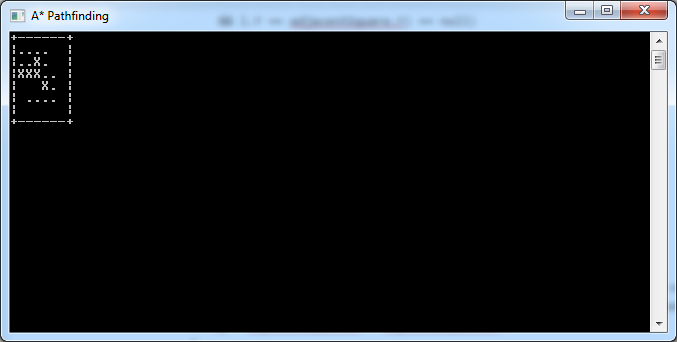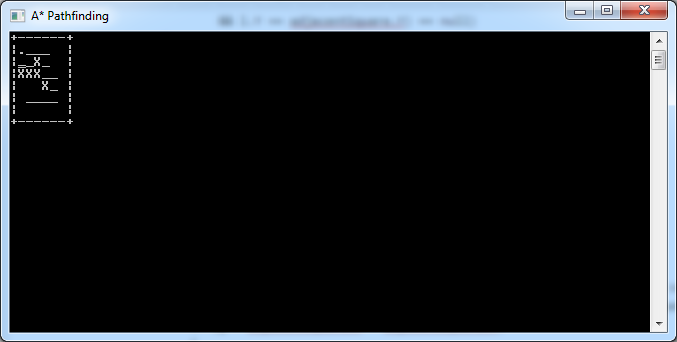# A* Pathfinding Example in C#

The A* pathfinding algorithm is one of the most popular ways of computing the shortest path between two points for game development. “Introduction to A* Pathfinding” by Johann Fradj illustrates the algorithm pretty nicely. I’ve written a C# implementation based on his example code, intended as nothing more than a learning exercise, and I am sharing it in this article. You can get the full source code directly from BitBucket; if you run it, you’ll see the algorithm run step by step.

Our A* algorithm will compute the shortest path between two points, A and B, on a small square grid of tiles. In order to really see it at work, we’ll introduce some obstacles, marked as X in the map below:

```            string[] map = new string[]
{
"+------+",
"|      |",
"|A X   |",
"|XXX   |",
"|   X  |",
"| B    |",
"|      |",
"+------+",
};
```

The A* algorithm needs to keep track of the tiles it’s visited (closed list) and the ones it’s considering (open list). For this we define a Location class:

```    class Location
{
public int X;
public int Y;
public int F;
public int G;
public int H;
public Location Parent;
}
```

Aside from the tile coordinates, each visited tile has three scores (F, G and H). The G score is the distance from the starting point, and the H score is the estimated distance from the destination (calculated as the city block distance). The F score is just G + H. For a full explanation of these values, read the reference article, “Introduction to A* Pathfinding“.

The H score is very simple to calculate – it’s just the horizontal and vertical distance from the current tile to the destination (ignoring any obstacles):

```        static int ComputeHScore(int x, int y, int targetX, int targetY)
{
return Math.Abs(targetX - x) + Math.Abs(targetY - y);
}
```

The Location class also has a Parent property. That’s used to store the previous tile, and it is needed to keep track of the path itself by the time the destination is reached.

Before we start the algorithm, we initialise some values:

```            Location current = null;
var start = new Location { X = 1, Y = 2 };
var target = new Location { X = 2, Y = 5 };
var openList = new List<Location>();
var closedList = new List<Location>();
int g = 0;

// start by adding the original position to the open list
```

The g value is the G-score; remember it’s the distance from the starting location to the current tile. We keep track of this simply by incrementing its value each time we move to a new tile.

We add the starting location to the open list, so that the algorithm can begin working. The algorithm itself runs in a loop:

```            while (openList.Count > 0)
{
// algorithm's logic goes here
}
```

For each iteration, the A* algorithm will retrieve the tile with the lowest F-score:

```                // get the square with the lowest F score
var lowest = openList.Min(l => l.F);
current = openList.First(l => l.F == lowest);
```

This is not necessarily the most efficient way of doing this, but works pretty well for this example. Also note that if there are multiple locations with the same F-score, it doesn’t really matter which is chosen (here we just take the first one) – again see “Introduction to A* Pathfinding” for details.

```                // add the current square to the closed list

// remove it from the open list
openList.Remove(current);
```

Here we add the current tile to the closed list, and remove it from the open list, to prevent it from being revisited in a subsequent iteration.

```                // show current square on the map
Console.SetCursorPosition(current.X, current.Y);
Console.Write('.');
Console.SetCursorPosition(current.X, current.Y);
```

The code above is not part of the A* algorithm. It simply marks the current tile with a dot and waits for a second so that you can follow the algorithm’s progress interactively.

```                // if we added the destination to the closed list, we've found a path
if (closedList.FirstOrDefault(l => l.X == target.X && l.Y == target.Y) != null)
break;
```

There are two conditions that allow the A* algorithm to terminate. One is that there are no more tiles in the open list to process, which would indicate that there is no path between A and B (we aren’t catering for this scenario in this example). The other is when the path is actually found, and that is catered for by the code above.

The rest of the algorithm evaluates adjacent tiles (i.e. the ones immediately one tile left, right, up and down from the current one):

```                var adjacentSquares = GetWalkableAdjacentSquares(current.X, current.Y, map);
g++;
```

```        static List<Location> GetWalkableAdjacentSquares(int x, int y, string[] map)
{
var proposedLocations = new List<Location>()
{
new Location { X = x, Y = y - 1 },
new Location { X = x, Y = y + 1 },
new Location { X = x - 1, Y = y },
new Location { X = x + 1, Y = y },
};

return proposedLocations.Where(
l => map[l.Y][l.X] == ' ' || map[l.Y][l.X] == 'B').ToList();
}
```

So back in the algorithm’s loop, we then have a loop over adjacent squares, computing their scores and adding them to the open list when it makes sense to do so:

```                foreach(var adjacentSquare in adjacentSquares)
{
// if this adjacent square is already in the closed list, ignore it
if (closedList.FirstOrDefault(l => l.X == adjacentSquare.X
&& l.Y == adjacentSquare.Y) != null)
continue;

// if it's not in the open list...
if (openList.FirstOrDefault(l => l.X == adjacentSquare.X
&& l.Y == adjacentSquare.Y) == null)
{
// compute its score, set the parent

// and add it to the open list
}
else
{
// test if using the current G score makes the adjacent square's F score
// lower, if yes update the parent because it means it's a better path
{
}
}
}
```

There’s some duplicate code in the if/else statement above, but I preferred to leave it that way as it’s more readable (which is more important here), especially if you’re following along with “Introduction to A* Pathfinding“.

That’s the last thing in the algorithm itself. So once it’s done, you can see the locations that were examined thanks to the dots drawn on the map:When it’s done, the program will run the following code, tracing its way back from destination to start by following the Parent property:

```            // assume path was found; let's show it
while (current != null)
{
Console.SetCursorPosition(current.X, current.Y);
Console.Write('_');
Console.SetCursorPosition(current.X, current.Y);
current = current.Parent;
}
```

This illustrates the actual shortest path by transforming the dots to underscores:You’ll notice that there is in fact a location that was examined but not used in the final path. That’s pretty normal, as A* may need to backtrack at times.

## 5 thoughts on “A* Pathfinding Example in C#”

1.chick hou says:

thanks for sharing!

2.kim dong hyun says:

great job! but i have a question
in your code the g value increase at every step(it’s never recalculated)
is it always OK to find shortest path even if g has not right value?

3.kim dong hyun says:

i think this code is more accurate..
g++ ;
=>
g = current.G + 1;